Publicité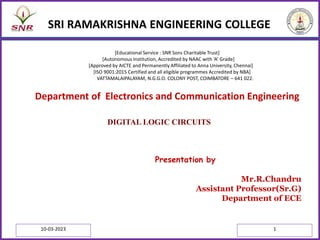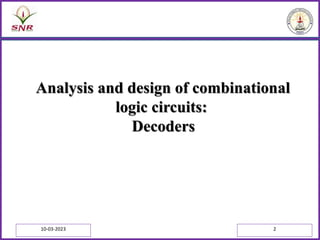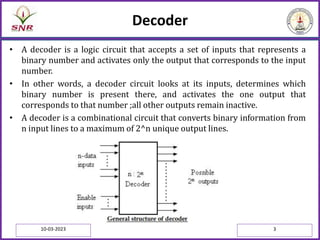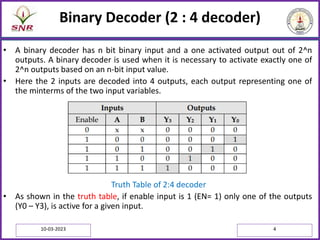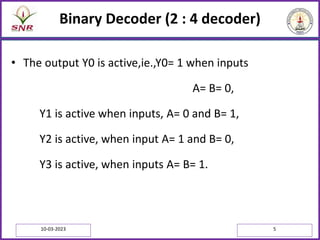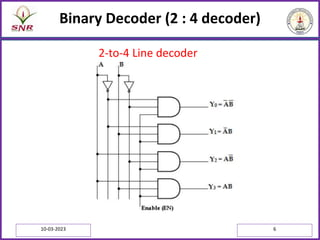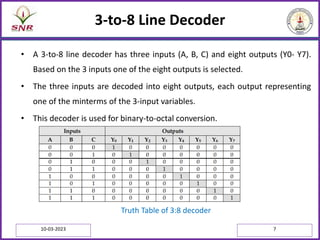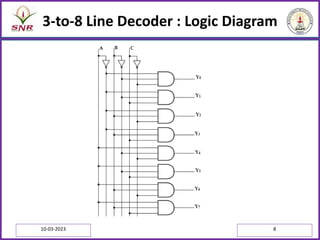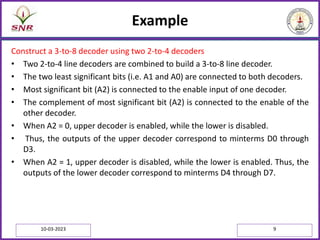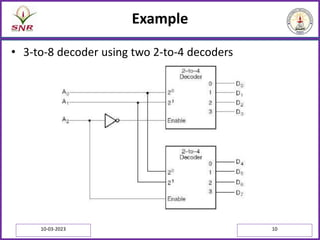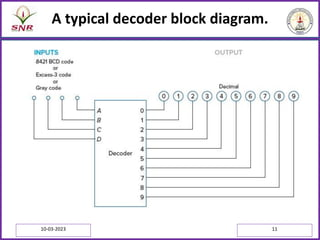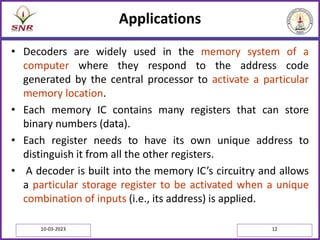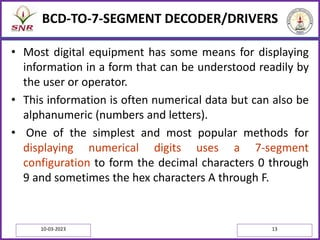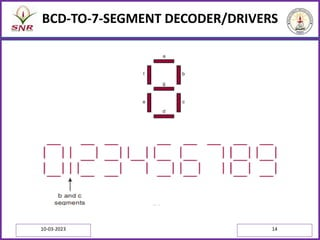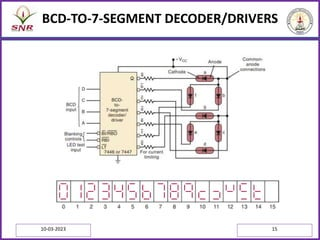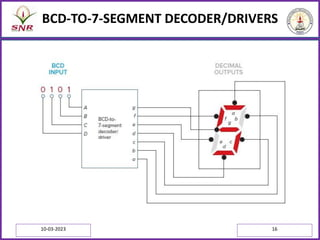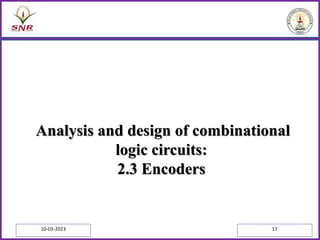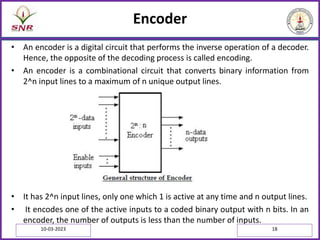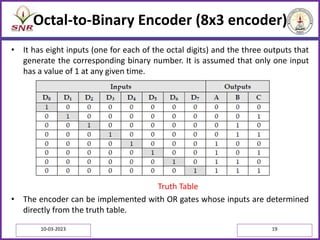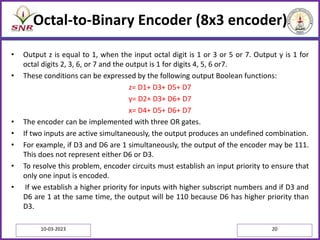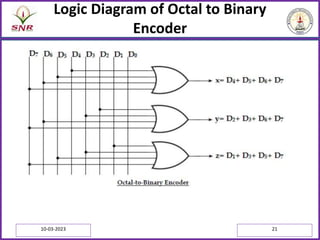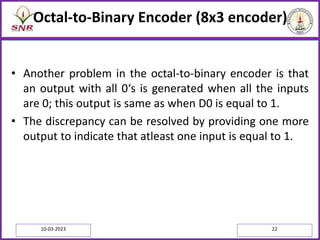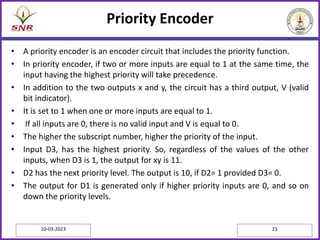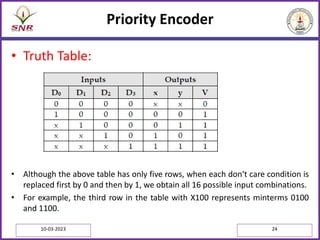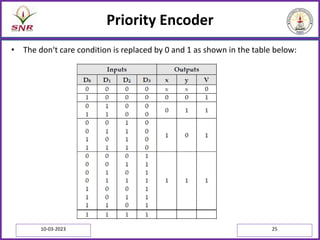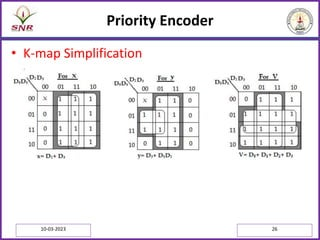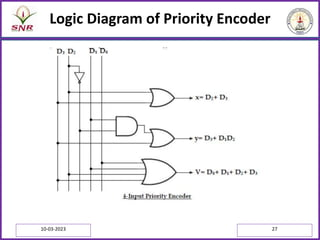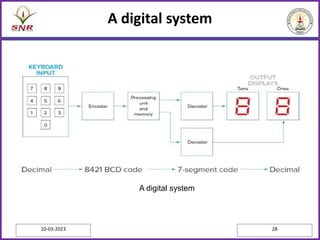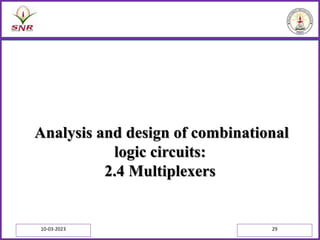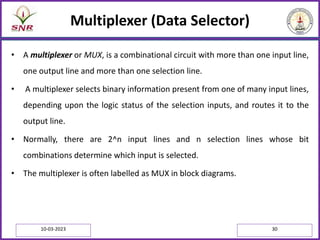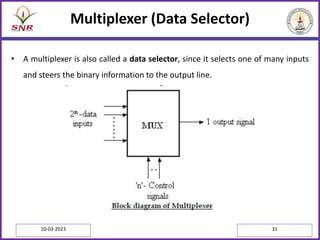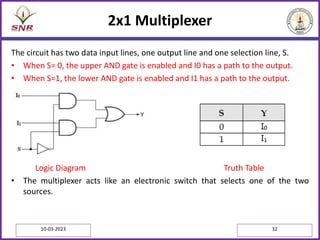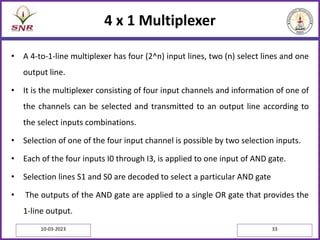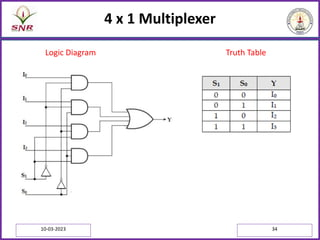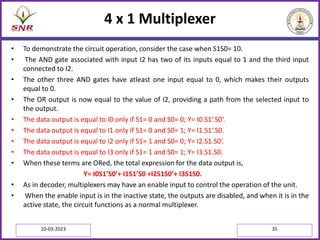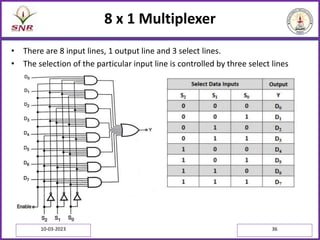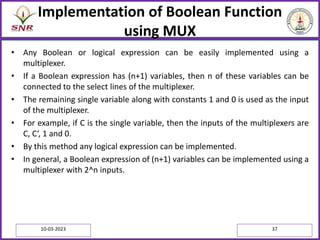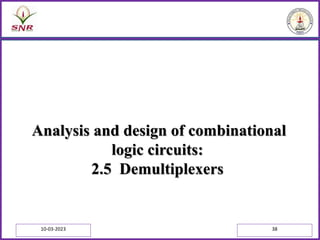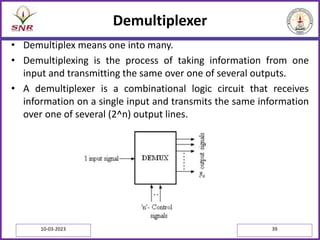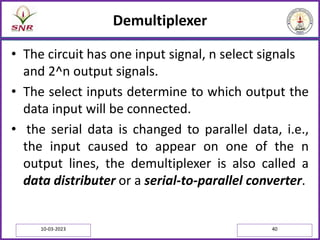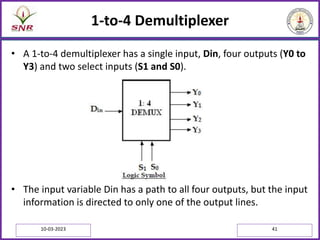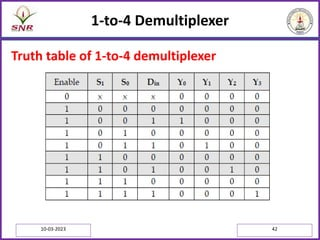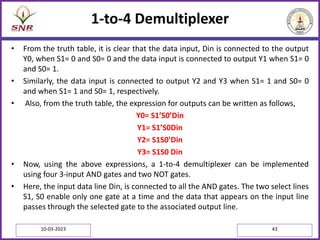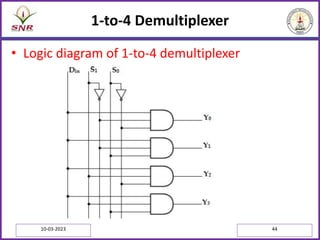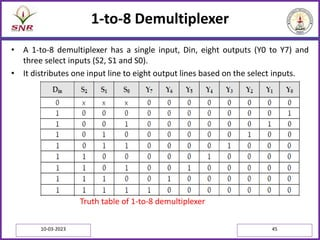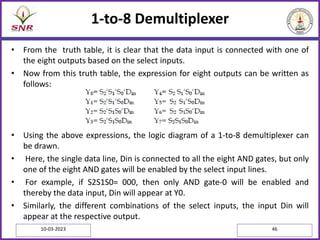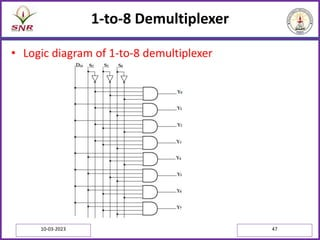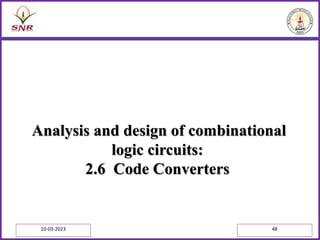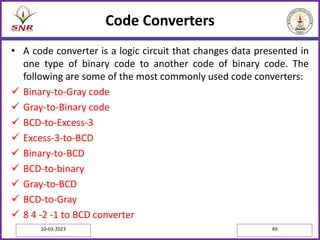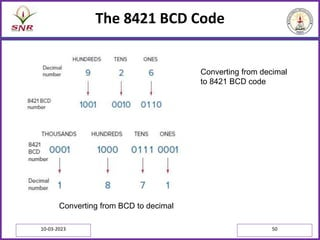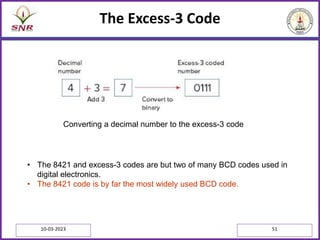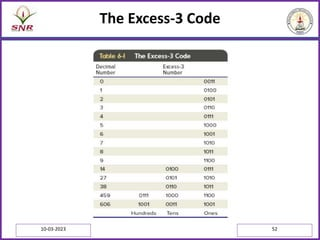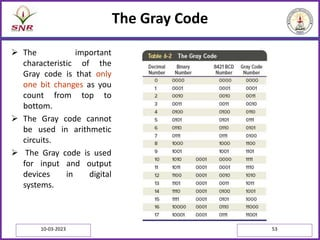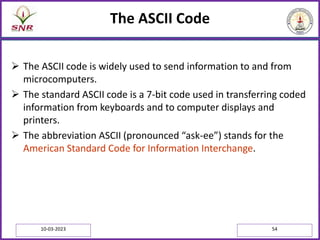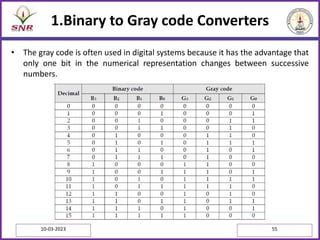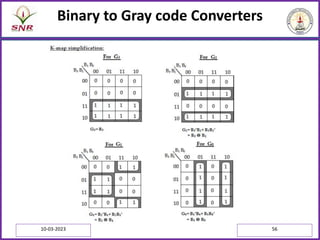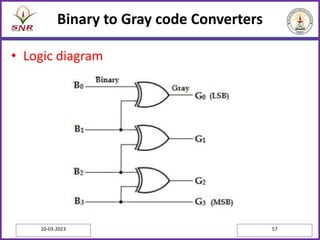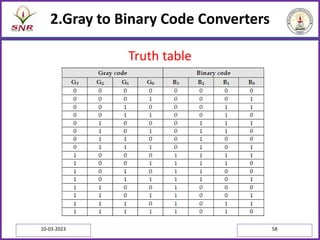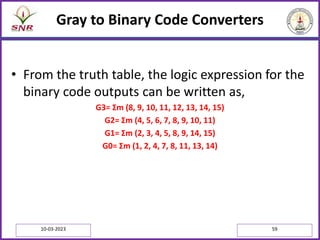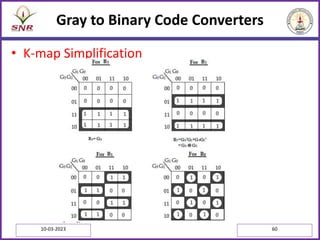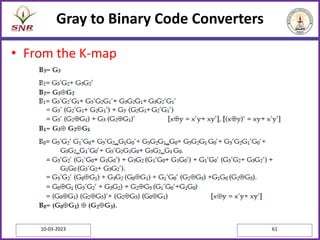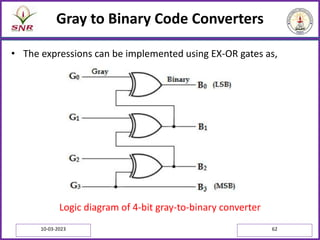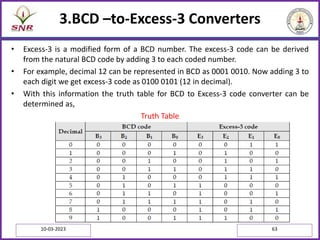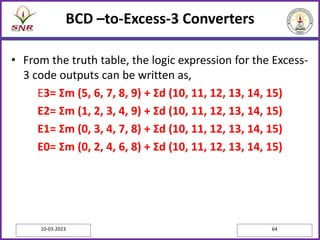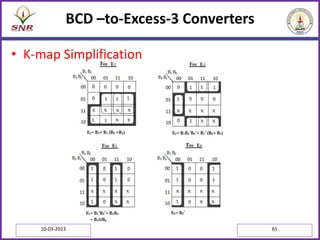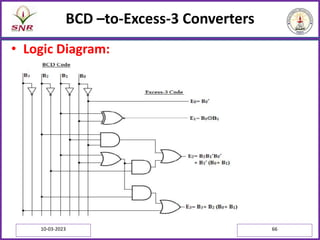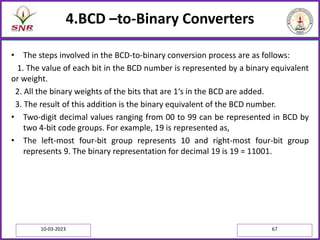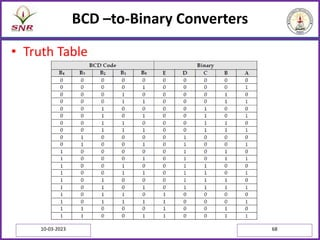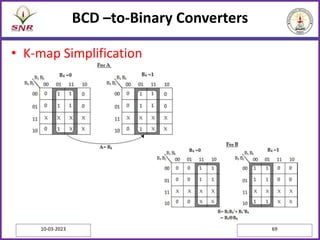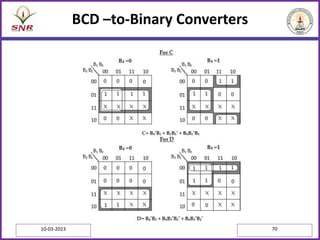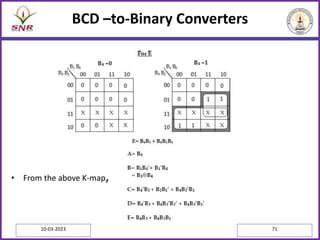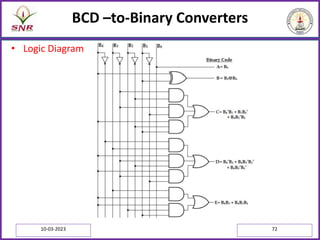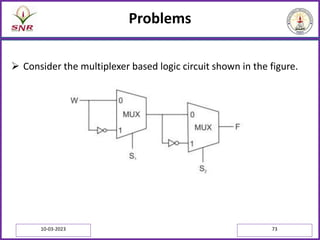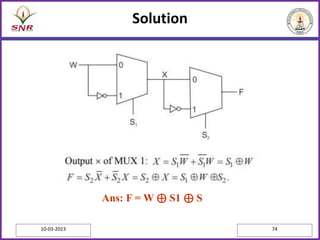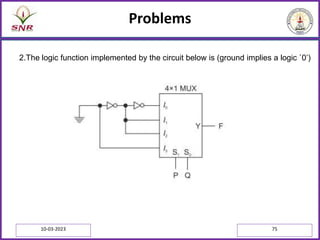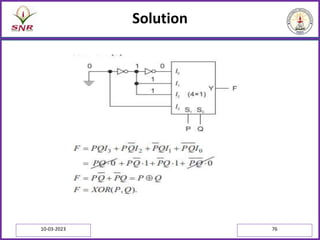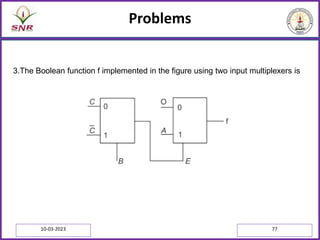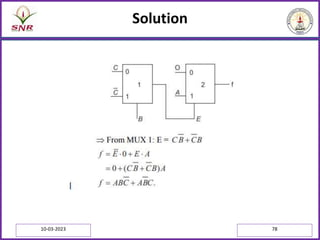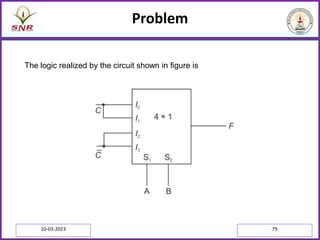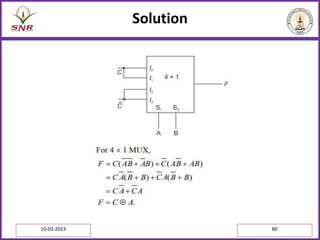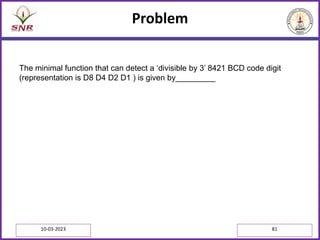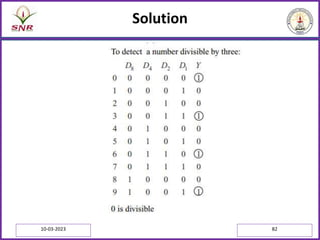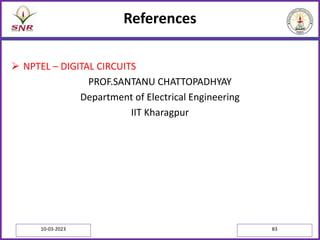1 sur 83
Publicité

### SESSION 2.ppt

1. SRI RAMAKRISHNA ENGINEERING COLLEGE Presentation by Mr.R.Chandru Assistant Professor(Sr.G) Department of ECE Department of Electronics and Communication Engineering [Educational Service : SNR Sons Charitable Trust] [Autonomous Institution, Accredited by NAAC with ‘A’ Grade] [Approved by AICTE and Permanently Affiliated to Anna University, Chennai] [ISO 9001:2015 Certified and all eligible programmes Accredited by NBA] VATTAMALAIPALAYAM, N.G.G.O. COLONY POST, COIMBATORE – 641 022. 10-03-2023 1 DIGITAL LOGIC CIRCUITS
2. Analysis and design of combinational logic circuits: Decoders 10-03-2023 2
3. Decoder • A decoder is a logic circuit that accepts a set of inputs that represents a binary number and activates only the output that corresponds to the input number. • In other words, a decoder circuit looks at its inputs, determines which binary number is present there, and activates the one output that corresponds to that number ;all other outputs remain inactive. • A decoder is a combinational circuit that converts binary information from n input lines to a maximum of 2^n unique output lines. 10-03-2023 3
4. Binary Decoder (2 : 4 decoder) • A binary decoder has n bit binary input and a one activated output out of 2^n outputs. A binary decoder is used when it is necessary to activate exactly one of 2^n outputs based on an n-bit input value. • Here the 2 inputs are decoded into 4 outputs, each output representing one of the minterms of the two input variables. Truth Table of 2:4 decoder • As shown in the truth table, if enable input is 1 (EN= 1) only one of the outputs (Y0 – Y3), is active for a given input. 10-03-2023 4
5. Binary Decoder (2 : 4 decoder) • The output Y0 is active,ie.,Y0= 1 when inputs A= B= 0, Y1 is active when inputs, A= 0 and B= 1, Y2 is active, when input A= 1 and B= 0, Y3 is active, when inputs A= B= 1. 10-03-2023 5
6. Binary Decoder (2 : 4 decoder) 2-to-4 Line decoder 10-03-2023 6
7. 3-to-8 Line Decoder • A 3-to-8 line decoder has three inputs (A, B, C) and eight outputs (Y0- Y7). Based on the 3 inputs one of the eight outputs is selected. • The three inputs are decoded into eight outputs, each output representing one of the minterms of the 3-input variables. • This decoder is used for binary-to-octal conversion. Truth Table of 3:8 decoder 10-03-2023 7
8. 3-to-8 Line Decoder : Logic Diagram 10-03-2023 8
9. Example Construct a 3-to-8 decoder using two 2-to-4 decoders • Two 2-to-4 line decoders are combined to build a 3-to-8 line decoder. • The two least significant bits (i.e. A1 and A0) are connected to both decoders. • Most significant bit (A2) is connected to the enable input of one decoder. • The complement of most significant bit (A2) is connected to the enable of the other decoder. • When A2 = 0, upper decoder is enabled, while the lower is disabled. • Thus, the outputs of the upper decoder correspond to minterms D0 through D3. • When A2 = 1, upper decoder is disabled, while the lower is enabled. Thus, the outputs of the lower decoder correspond to minterms D4 through D7. 10-03-2023 9
10. Example • 3-to-8 decoder using two 2-to-4 decoders 10-03-2023 10
11. A typical decoder block diagram. 10-03-2023 11
12. Applications • Decoders are widely used in the memory system of a computer where they respond to the address code generated by the central processor to activate a particular memory location. • Each memory IC contains many registers that can store binary numbers (data). • Each register needs to have its own unique address to distinguish it from all the other registers. • A decoder is built into the memory IC’s circuitry and allows a particular storage register to be activated when a unique combination of inputs (i.e., its address) is applied. 10-03-2023 12
13. BCD-TO-7-SEGMENT DECODER/DRIVERS • Most digital equipment has some means for displaying information in a form that can be understood readily by the user or operator. • This information is often numerical data but can also be alphanumeric (numbers and letters). • One of the simplest and most popular methods for displaying numerical digits uses a 7-segment configuration to form the decimal characters 0 through 9 and sometimes the hex characters A through F. 10-03-2023 13
14. BCD-TO-7-SEGMENT DECODER/DRIVERS 10-03-2023 14
15. BCD-TO-7-SEGMENT DECODER/DRIVERS 10-03-2023 15
16. BCD-TO-7-SEGMENT DECODER/DRIVERS 10-03-2023 16
17. Analysis and design of combinational logic circuits: 2.3 Encoders 10-03-2023 17
18. Encoder • An encoder is a digital circuit that performs the inverse operation of a decoder. Hence, the opposite of the decoding process is called encoding. • An encoder is a combinational circuit that converts binary information from 2^n input lines to a maximum of n unique output lines. • It has 2^n input lines, only one which 1 is active at any time and n output lines. • It encodes one of the active inputs to a coded binary output with n bits. In an encoder, the number of outputs is less than the number of inputs. 10-03-2023 18
19. Octal-to-Binary Encoder (8x3 encoder) • It has eight inputs (one for each of the octal digits) and the three outputs that generate the corresponding binary number. It is assumed that only one input has a value of 1 at any given time. Truth Table • The encoder can be implemented with OR gates whose inputs are determined directly from the truth table. 10-03-2023 19
20. Octal-to-Binary Encoder (8x3 encoder) • Output z is equal to 1, when the input octal digit is 1 or 3 or 5 or 7. Output y is 1 for octal digits 2, 3, 6, or 7 and the output is 1 for digits 4, 5, 6 or7. • These conditions can be expressed by the following output Boolean functions: z= D1+ D3+ D5+ D7 y= D2+ D3+ D6+ D7 x= D4+ D5+ D6+ D7 • The encoder can be implemented with three OR gates. • If two inputs are active simultaneously, the output produces an undefined combination. • For example, if D3 and D6 are 1 simultaneously, the output of the encoder may be 111. This does not represent either D6 or D3. • To resolve this problem, encoder circuits must establish an input priority to ensure that only one input is encoded. • If we establish a higher priority for inputs with higher subscript numbers and if D3 and D6 are 1 at the same time, the output will be 110 because D6 has higher priority than D3. 10-03-2023 20
21. Logic Diagram of Octal to Binary Encoder 10-03-2023 21
22. Octal-to-Binary Encoder (8x3 encoder) • Another problem in the octal-to-binary encoder is that an output with all 0‘s is generated when all the inputs are 0; this output is same as when D0 is equal to 1. • The discrepancy can be resolved by providing one more output to indicate that atleast one input is equal to 1. 10-03-2023 22
23. Priority Encoder • A priority encoder is an encoder circuit that includes the priority function. • In priority encoder, if two or more inputs are equal to 1 at the same time, the input having the highest priority will take precedence. • In addition to the two outputs x and y, the circuit has a third output, V (valid bit indicator). • It is set to 1 when one or more inputs are equal to 1. • If all inputs are 0, there is no valid input and V is equal to 0. • The higher the subscript number, higher the priority of the input. • Input D3, has the highest priority. So, regardless of the values of the other inputs, when D3 is 1, the output for xy is 11. • D2 has the next priority level. The output is 10, if D2= 1 provided D3= 0. • The output for D1 is generated only if higher priority inputs are 0, and so on down the priority levels. 10-03-2023 23
24. Priority Encoder • Truth Table: • Although the above table has only five rows, when each don‘t care condition is replaced first by 0 and then by 1, we obtain all 16 possible input combinations. • For example, the third row in the table with X100 represents minterms 0100 and 1100. 10-03-2023 24
25. Priority Encoder • The don‘t care condition is replaced by 0 and 1 as shown in the table below: 10-03-2023 25
26. Priority Encoder • K-map Simplification 10-03-2023 26
27. Logic Diagram of Priority Encoder 10-03-2023 27
28. A digital system 10-03-2023 28 A digital system
29. Analysis and design of combinational logic circuits: 2.4 Multiplexers 10-03-2023 29
30. Multiplexer (Data Selector) • A multiplexer or MUX, is a combinational circuit with more than one input line, one output line and more than one selection line. • A multiplexer selects binary information present from one of many input lines, depending upon the logic status of the selection inputs, and routes it to the output line. • Normally, there are 2^n input lines and n selection lines whose bit combinations determine which input is selected. • The multiplexer is often labelled as MUX in block diagrams. 10-03-2023 30
31. Multiplexer (Data Selector) • A multiplexer is also called a data selector, since it selects one of many inputs and steers the binary information to the output line. 10-03-2023 31
32. 2x1 Multiplexer The circuit has two data input lines, one output line and one selection line, S. • When S= 0, the upper AND gate is enabled and I0 has a path to the output. • When S=1, the lower AND gate is enabled and I1 has a path to the output. Logic Diagram Truth Table • The multiplexer acts like an electronic switch that selects one of the two sources. 10-03-2023 32
33. 4 x 1 Multiplexer • A 4-to-1-line multiplexer has four (2^n) input lines, two (n) select lines and one output line. • It is the multiplexer consisting of four input channels and information of one of the channels can be selected and transmitted to an output line according to the select inputs combinations. • Selection of one of the four input channel is possible by two selection inputs. • Each of the four inputs I0 through I3, is applied to one input of AND gate. • Selection lines S1 and S0 are decoded to select a particular AND gate • The outputs of the AND gate are applied to a single OR gate that provides the 1-line output. 10-03-2023 33
34. 4 x 1 Multiplexer Logic Diagram Truth Table 10-03-2023 34
35. 4 x 1 Multiplexer • To demonstrate the circuit operation, consider the case when S1S0= 10. • The AND gate associated with input I2 has two of its inputs equal to 1 and the third input connected to I2. • The other three AND gates have atleast one input equal to 0, which makes their outputs equal to 0. • The OR output is now equal to the value of I2, providing a path from the selected input to the output. • The data output is equal to I0 only if S1= 0 and S0= 0; Y= I0.S1‘.S0‘. • The data output is equal to I1 only if S1= 0 and S0= 1; Y= I1.S1‘.S0. • The data output is equal to I2 only if S1= 1 and S0= 0; Y= I2.S1.S0‘. • The data output is equal to I3 only if S1= 1 and S0= 1; Y= I3.S1.S0. • When these terms are ORed, the total expression for the data output is, Y= I0S1’S0’+ I1S1’S0 +I2S1S0’+ I3S1S0. • As in decoder, multiplexers may have an enable input to control the operation of the unit. • When the enable input is in the inactive state, the outputs are disabled, and when it is in the active state, the circuit functions as a normal multiplexer. 10-03-2023 35
36. 8 x 1 Multiplexer • There are 8 input lines, 1 output line and 3 select lines. • The selection of the particular input line is controlled by three select lines Logic Diagram Truth Table 10-03-2023 36
37. Implementation of Boolean Function using MUX • Any Boolean or logical expression can be easily implemented using a multiplexer. • If a Boolean expression has (n+1) variables, then n of these variables can be connected to the select lines of the multiplexer. • The remaining single variable along with constants 1 and 0 is used as the input of the multiplexer. • For example, if C is the single variable, then the inputs of the multiplexers are C, C‘, 1 and 0. • By this method any logical expression can be implemented. • In general, a Boolean expression of (n+1) variables can be implemented using a multiplexer with 2^n inputs. 10-03-2023 37
38. Analysis and design of combinational logic circuits: 2.5 Demultiplexers 10-03-2023 38
39. Demultiplexer • Demultiplex means one into many. • Demultiplexing is the process of taking information from one input and transmitting the same over one of several outputs. • A demultiplexer is a combinational logic circuit that receives information on a single input and transmits the same information over one of several (2^n) output lines. 10-03-2023 39
40. Demultiplexer • The circuit has one input signal, n select signals and 2^n output signals. • The select inputs determine to which output the data input will be connected. • the serial data is changed to parallel data, i.e., the input caused to appear on one of the n output lines, the demultiplexer is also called a data distributer or a serial-to-parallel converter. 10-03-2023 40
41. 1-to-4 Demultiplexer • A 1-to-4 demultiplexer has a single input, Din, four outputs (Y0 to Y3) and two select inputs (S1 and S0). • The input variable Din has a path to all four outputs, but the input information is directed to only one of the output lines. 10-03-2023 41
42. 1-to-4 Demultiplexer Truth table of 1-to-4 demultiplexer 10-03-2023 42
43. 1-to-4 Demultiplexer • From the truth table, it is clear that the data input, Din is connected to the output Y0, when S1= 0 and S0= 0 and the data input is connected to output Y1 when S1= 0 and S0= 1. • Similarly, the data input is connected to output Y2 and Y3 when S1= 1 and S0= 0 and when S1= 1 and S0= 1, respectively. • Also, from the truth table, the expression for outputs can be written as follows, Y0= S1’S0’Din Y1= S1’S0Din Y2= S1S0’Din Y3= S1S0 Din • Now, using the above expressions, a 1-to-4 demultiplexer can be implemented using four 3-input AND gates and two NOT gates. • Here, the input data line Din, is connected to all the AND gates. The two select lines S1, S0 enable only one gate at a time and the data that appears on the input line passes through the selected gate to the associated output line. 10-03-2023 43
44. 1-to-4 Demultiplexer • Logic diagram of 1-to-4 demultiplexer 10-03-2023 44
45. 1-to-8 Demultiplexer • A 1-to-8 demultiplexer has a single input, Din, eight outputs (Y0 to Y7) and three select inputs (S2, S1 and S0). • It distributes one input line to eight output lines based on the select inputs. Truth table of 1-to-8 demultiplexer 10-03-2023 45
46. 1-to-8 Demultiplexer • From the truth table, it is clear that the data input is connected with one of the eight outputs based on the select inputs. • Now from this truth table, the expression for eight outputs can be written as follows: • Using the above expressions, the logic diagram of a 1-to-8 demultiplexer can be drawn. • Here, the single data line, Din is connected to all the eight AND gates, but only one of the eight AND gates will be enabled by the select input lines. • For example, if S2S1S0= 000, then only AND gate-0 will be enabled and thereby the data input, Din will appear at Y0. • Similarly, the different combinations of the select inputs, the input Din will appear at the respective output. 10-03-2023 46
47. 1-to-8 Demultiplexer • Logic diagram of 1-to-8 demultiplexer 10-03-2023 47
48. Analysis and design of combinational logic circuits: 2.6 Code Converters 10-03-2023 48
49. Code Converters • A code converter is a logic circuit that changes data presented in one type of binary code to another code of binary code. The following are some of the most commonly used code converters:  Binary-to-Gray code  Gray-to-Binary code  BCD-to-Excess-3  Excess-3-to-BCD  Binary-to-BCD  BCD-to-binary  Gray-to-BCD  BCD-to-Gray  8 4 -2 -1 to BCD converter 10-03-2023 49
50. The 8421 BCD Code 10-03-2023 50 Converting from decimal to 8421 BCD code Converting from BCD to decimal
51. The Excess-3 Code 10-03-2023 51 Converting a decimal number to the excess-3 code • The 8421 and excess-3 codes are but two of many BCD codes used in digital electronics. • The 8421 code is by far the most widely used BCD code.
52. The Excess-3 Code 10-03-2023 52
53. The Gray Code  The important characteristic of the Gray code is that only one bit changes as you count from top to bottom.  The Gray code cannot be used in arithmetic circuits.  The Gray code is used for input and output devices in digital systems. 10-03-2023 53
54. The ASCII Code  The ASCII code is widely used to send information to and from microcomputers.  The standard ASCII code is a 7-bit code used in transferring coded information from keyboards and to computer displays and printers.  The abbreviation ASCII (pronounced “ask-ee”) stands for the American Standard Code for Information Interchange. 10-03-2023 54
55. 1.Binary to Gray code Converters • The gray code is often used in digital systems because it has the advantage that only one bit in the numerical representation changes between successive numbers. Truth Table 10-03-2023 55
56. Binary to Gray code Converters 10-03-2023 56
57. Binary to Gray code Converters • Logic diagram 10-03-2023 57
58. 2.Gray to Binary Code Converters Truth table 10-03-2023 58
59. Gray to Binary Code Converters • From the truth table, the logic expression for the binary code outputs can be written as, G3= Σm (8, 9, 10, 11, 12, 13, 14, 15) G2= Σm (4, 5, 6, 7, 8, 9, 10, 11) G1= Σm (2, 3, 4, 5, 8, 9, 14, 15) G0= Σm (1, 2, 4, 7, 8, 11, 13, 14) 10-03-2023 59
60. Gray to Binary Code Converters • K-map Simplification 10-03-2023 60
61. Gray to Binary Code Converters • From the K-map 10-03-2023 61
62. Gray to Binary Code Converters • The expressions can be implemented using EX-OR gates as, Logic diagram of 4-bit gray-to-binary converter 10-03-2023 62
63. 3.BCD –to-Excess-3 Converters • Excess-3 is a modified form of a BCD number. The excess-3 code can be derived from the natural BCD code by adding 3 to each coded number. • For example, decimal 12 can be represented in BCD as 0001 0010. Now adding 3 to each digit we get excess-3 code as 0100 0101 (12 in decimal). • With this information the truth table for BCD to Excess-3 code converter can be determined as, Truth Table 10-03-2023 63
64. BCD –to-Excess-3 Converters • From the truth table, the logic expression for the Excess- 3 code outputs can be written as, E3= Σm (5, 6, 7, 8, 9) + Σd (10, 11, 12, 13, 14, 15) E2= Σm (1, 2, 3, 4, 9) + Σd (10, 11, 12, 13, 14, 15) E1= Σm (0, 3, 4, 7, 8) + Σd (10, 11, 12, 13, 14, 15) E0= Σm (0, 2, 4, 6, 8) + Σd (10, 11, 12, 13, 14, 15) 10-03-2023 64
65. BCD –to-Excess-3 Converters • K-map Simplification 10-03-2023 65
66. BCD –to-Excess-3 Converters • Logic Diagram: 10-03-2023 66
67. 4.BCD –to-Binary Converters • The steps involved in the BCD-to-binary conversion process are as follows: 1. The value of each bit in the BCD number is represented by a binary equivalent or weight. 2. All the binary weights of the bits that are 1‘s in the BCD are added. 3. The result of this addition is the binary equivalent of the BCD number. • Two-digit decimal values ranging from 00 to 99 can be represented in BCD by two 4-bit code groups. For example, 19 is represented as, • The left-most four-bit group represents 10 and right-most four-bit group represents 9. The binary representation for decimal 19 is 19 = 11001. 10-03-2023 67
68. BCD –to-Binary Converters • Truth Table 10-03-2023 68
69. BCD –to-Binary Converters • K-map Simplification 10-03-2023 69
70. BCD –to-Binary Converters 10-03-2023 70
71. BCD –to-Binary Converters • From the above K-map, 10-03-2023 71
72. BCD –to-Binary Converters • Logic Diagram 10-03-2023 72
73. Problems  Consider the multiplexer based logic circuit shown in the figure. 10-03-2023 73
74. Solution 10-03-2023 74 Ans: F = W ⊕ S1 ⊕ S
75. Problems 10-03-2023 75 2.The logic function implemented by the circuit below is (ground implies a logic `0’)
76. Solution 10-03-2023 76
77. Problems 10-03-2023 77 3.The Boolean function f implemented in the figure using two input multiplexers is
78. Solution 10-03-2023 78
79. Problem 10-03-2023 79 The logic realized by the circuit shown in figure is
80. Solution 10-03-2023 80
81. Problem 10-03-2023 81 The minimal function that can detect a ‘divisible by 3’ 8421 BCD code digit (representation is D8 D4 D2 D1 ) is given by_________
82. Solution 10-03-2023 82
83. References  NPTEL – DIGITAL CIRCUITS PROF.SANTANU CHATTOPADHYAY Department of Electrical Engineering IIT Kharagpur 10-03-2023 83
Publicité